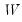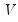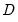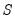User’s Guide : Basic Data Analysis : Series : Wavelet Objects

Wavelet Objects
EViews offers series views that use wavelet analysis to perform a variety of useful operations:
Wavelet transforms
Variance decomposition
Outlier detection
Thresholding (denoising)
Click on Proc/Make Wavelet Objects... to display the dialogThe Wavelet Procedure dropdown lets you choose between the four different types of wavelet procedures: Transform, Variance decomposition, Outlier detection, Threshold (Denoising). As you select different procedures the remainder of the dialog will change to reflect the options available for the procedure.
New to this dialog are the Output prefix options where you will provide base names to be used in creating the output vectors.
Different results are saved in the different cases:
1. Transform
if DWT or MODWT the results will contain the wavelet coefficientsand(vectors). The strings “_w” and “_v” followed by the scale level will be appended to the base name.
if DWT MRA or MODWT MRA the results will containand(vectors). The strings “_d” and “_s” followed by the scale level will be appended to the base name.
Thus, if the base name is “X”, the DWT wavelet coefficients and scaling will be saved in X_W1, X_V1, etc.
2. Variance decomposition
variance at each scale (single vector). The string “_var” will be appended to the base name.
3. Threshold
thresholded signal (vector). The string “_sig” followed by “_th”, will be appended to the base name.
thresholded noise (vector). The string “_res” followed by “_th”, will be appended to the base name.
thresholdedat each scale (vectors). The string “_w” followed by the scale level, followed by “_th”, will be appended to the base name.
regularat each scale (vectors). The string “_v” followed by the scale level will be appended to the base name.
Thus, if the base name is “X”, the results will be saved into X_SIG_TH and X_RES_TH, and vectors X_W1_TH, X_V1_TH, etc.
4. Outliers
outliers at each scale with outlying observation indices as contents (vectors). The string “_ol” followed by the scale level will be appended to the base name.
Thus, if the base name is “X”, the results will be saved into X_OLR1, X_OLR2, etc.
For extensive discussion of each of these procedures, results, and the associated options, see “Performing Wavelet Analysis in EViews”.